## Machine Learning and Artificial Intelligence for computational nuclear data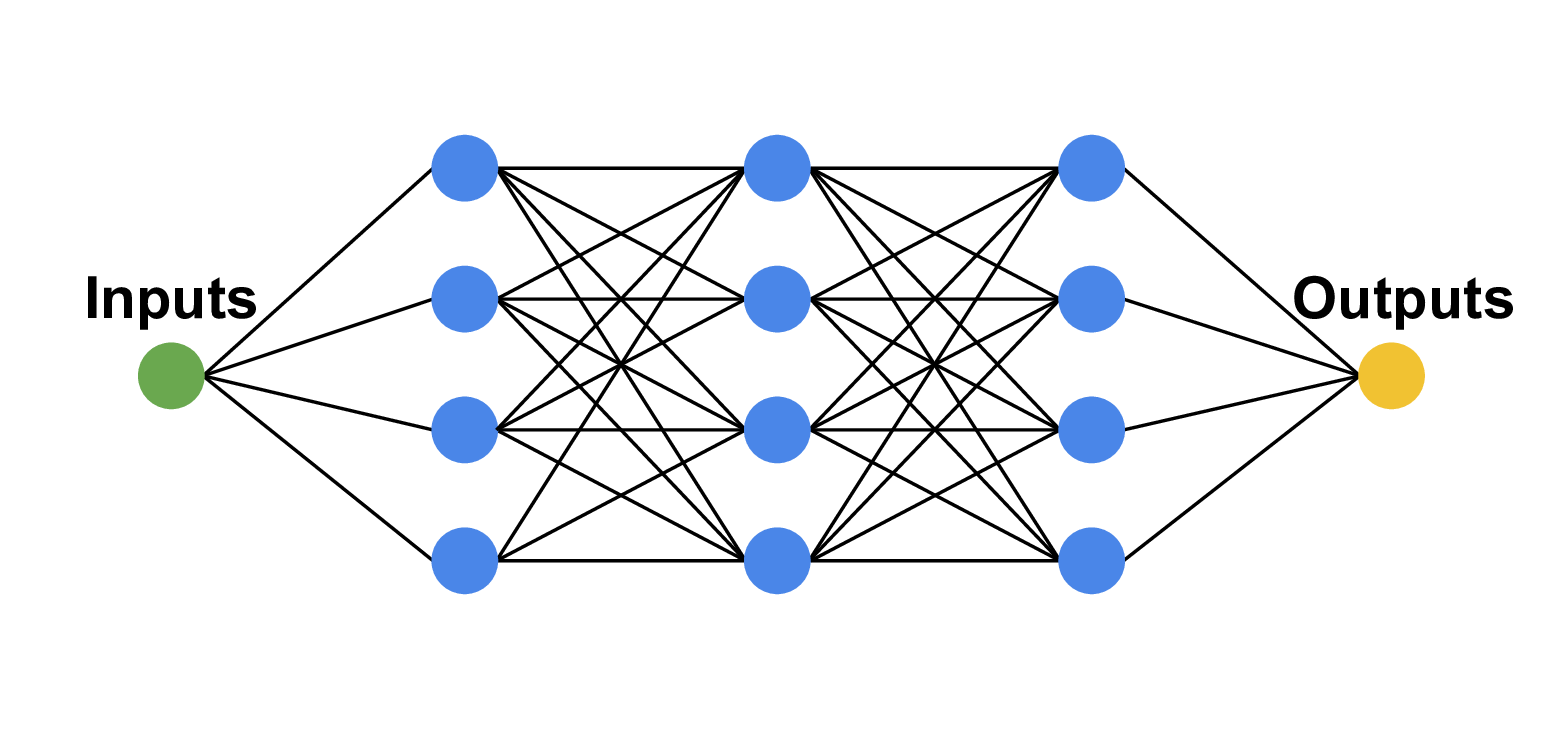LA-UR-21-30576

### Matthew Mumpower

IAEA AI4Atoms Workshop

Monday October 25$^{th}$ 2021Nuclear Data Team
Theoretical DivisionLos Alamos National Laboratory Caveat

The submitted materials have been authored by an employee or employees of Triad National Security, LLC (Triad) under contract with the U.S. Department of Energy/National Nuclear Security Administration (DOE/NNSA).

Accordingly, the U.S. Government retains an irrevocable, nonexclusive, royaltyfree license to publish, translate, reproduce, use, or dispose of the published form of the work and to authorize others to do the same for U.S. Government purposes.

## Nuclear Data is Ubiquitous in modern applications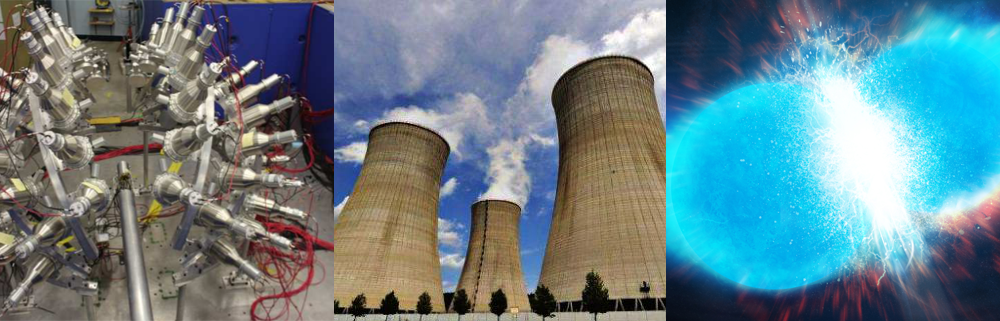Example: fission yields are needed for a variety of applications and cutting-edge science

Industrial applications: simulation of reactors, fuel cycles, waste management

Experiments: backgrounds, isotope production with radioactive ion beams (fragmentation)

Science applications: nucleosynthesis, light curve observations

Other Applications: national security, nonproliferation, nuclear forensics

Figure: Chi-Nu detector (left), Reactor cooling towers (middle), Neutron star merger (right)

## Nuclear data from a forward problem perspective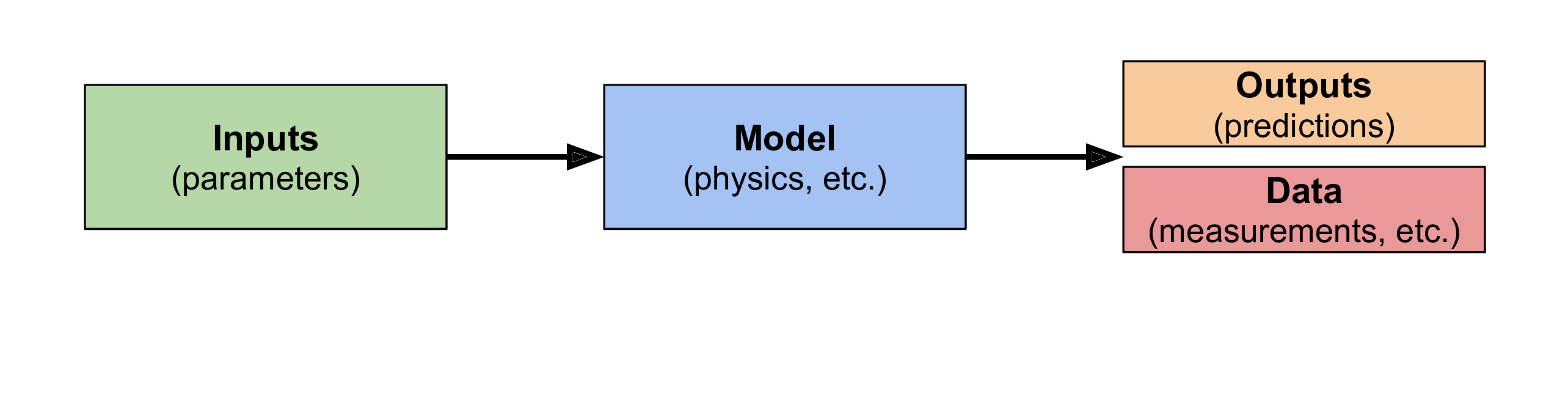When we model nuclear data we think of it as a 'forward' problem

We start with our model and parameters and try to match measurements / observations

This is an extremely successful approach and is precisely how nuclear theory modeling for nuclear data works

Mathematically: $f(\vec{\boldsymbol{x}}) = \vec{\boldsymbol{y}}$

Where $f$ is our model, $\vec{\boldsymbol{x}}$ are the parameters for the model and $\vec{\boldsymbol{y}}$ are the predictions

Figure by Mumpower

## Forward problem... problems...There can be many challenges with this approach

For instance, how do we update our model if we don't match data?

This can be challenging

We don't always know what modifications are required or the physics could be very hard if not impossible to model given current computational limitations (e.g. many-body problem)

Treating this as an 'inverse problem' provides an alternative approach...

Figure by Mumpower

## Nuclear data from an inverse problem perspective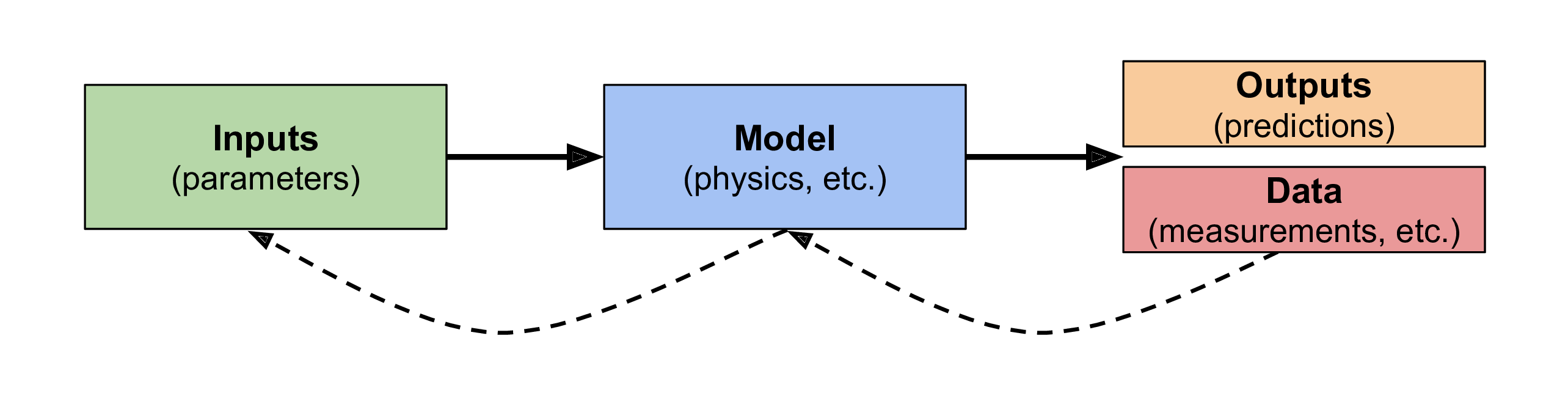We can then try to figure out what models may match relevant observations

This approach allows us to vary and optimize the model!

Mathematically, still: $f(\vec{\boldsymbol{x}}) = \vec{\boldsymbol{y}}$; but we want to now find $f$; fixing $\vec{\boldsymbol{x}}$

Machine learning and artificial intelligence algorithms are ideal for this class of problems...

Figure by Mumpower

## An example of how this works (from my life)

My son Zachary has been very keen on learning his letters from an early age

Letters are well known (this represents the data we want to match to)

But the model (being able to draw - eventually letters) must be developed, practiced and finally optimized

This is a complex, iterative process - it takes times to find '$f$'!

## An example of how this works (from my life)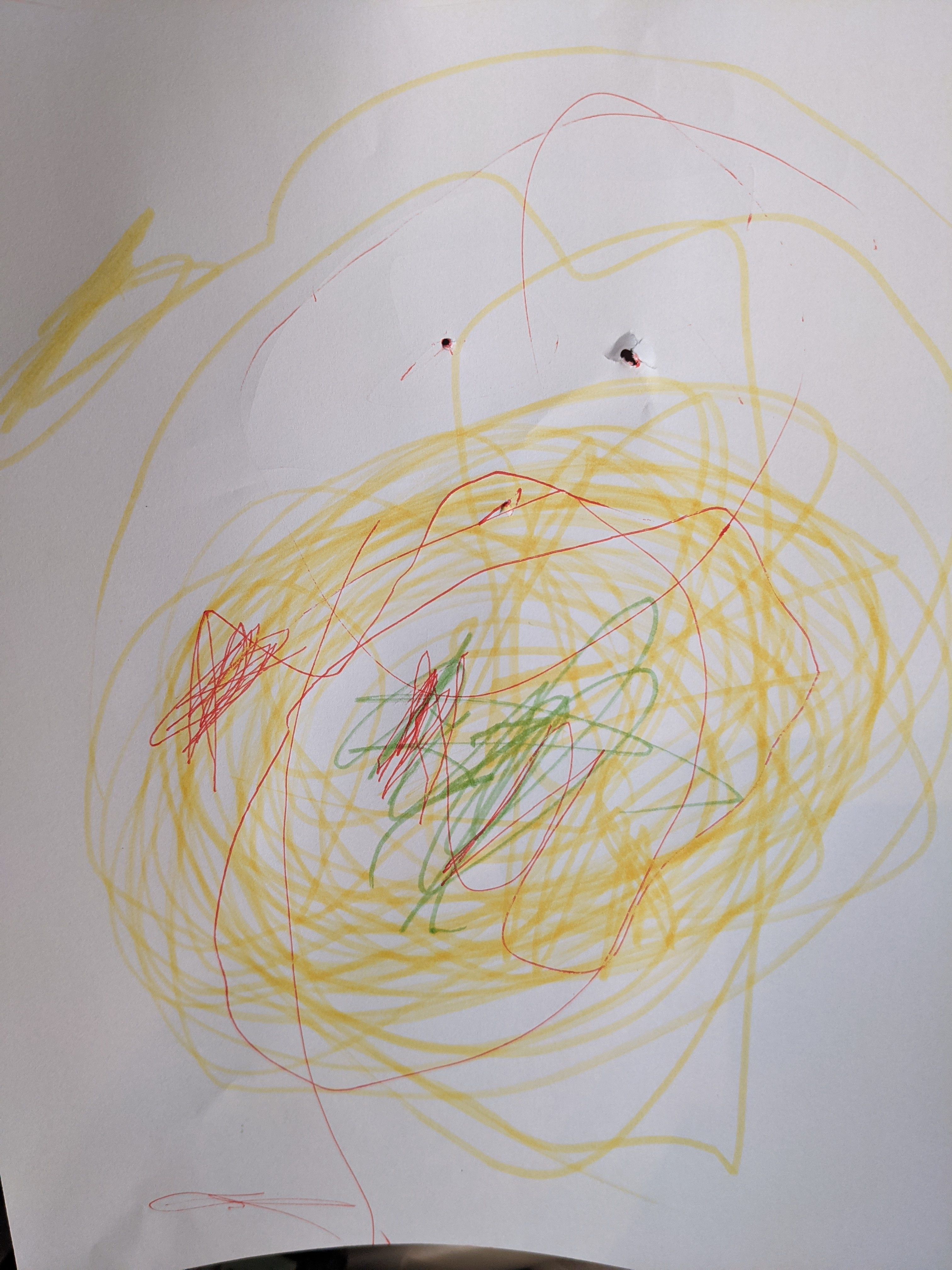Example from 1 years old; random drawing episode; time taken: 10 minutes

Drawing by Z. R. Mumpower

## An example of how this works (from my life)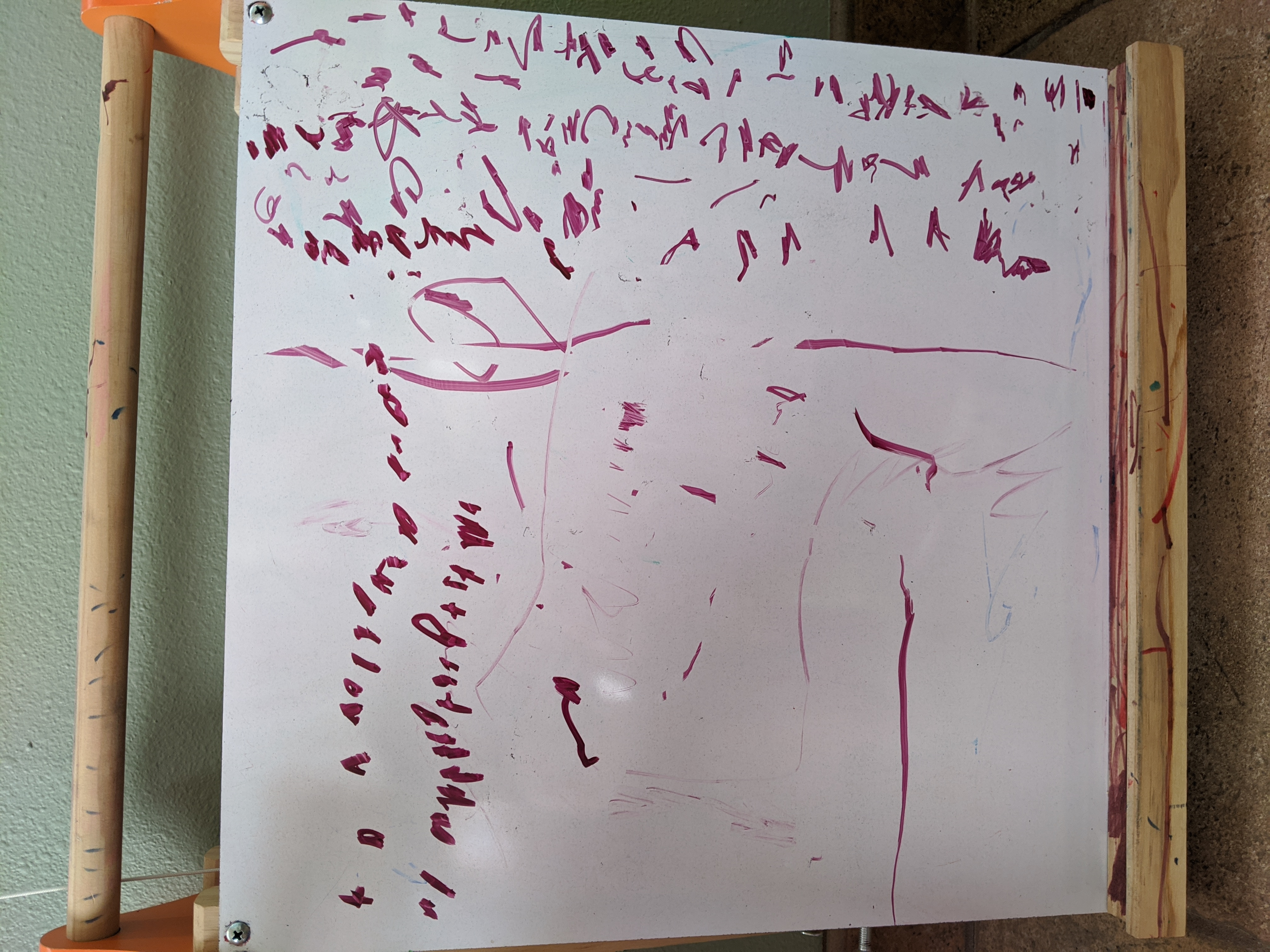Example from 2.5 years old; first ever attempt to draw letters / alphabet; time taken: 2 hours!

Drawing by Z. R. Mumpower

## An example of how this works (from my life)Example from 3.5 years old; drawing scenery; time taken: 10 minutes

Drawing by Z. R. Mumpower

## An example of how this works (from my life)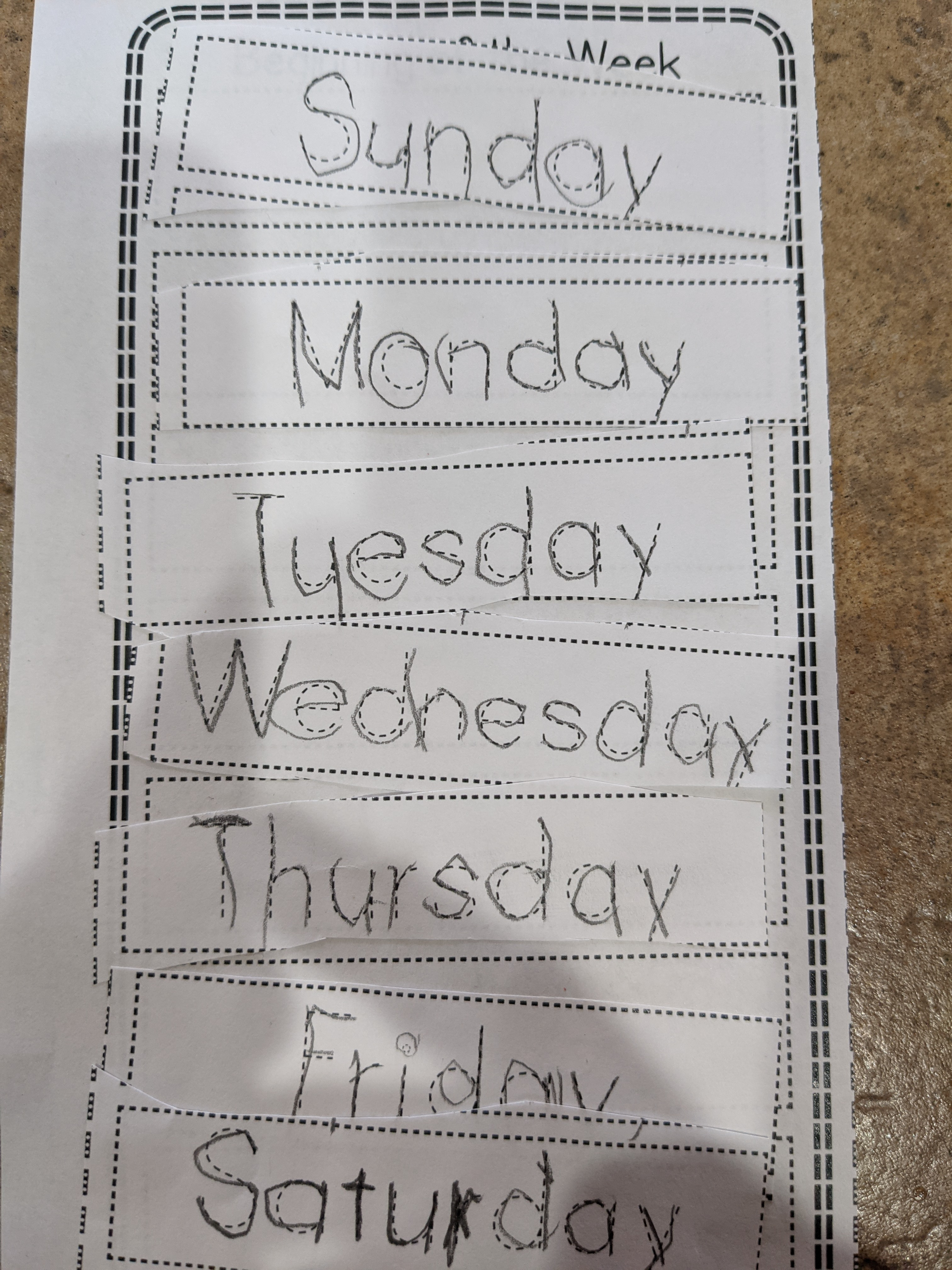Example from 4 years old; tracing the days of the week; time taken: 30 minutes

Drawing by Z. R. Mumpower

## Let's apply this idea to nuclear data...

A relatively easy choice is a scalar property; how about atomic binding energies (masses)?

Recall: $f(\vec{\boldsymbol{x}}) = \vec{\boldsymbol{y}}$

$f$ will be a neural network (a model that can change)

$\vec{\boldsymbol{y}_{obs}}$ are the observations (e.g. Atomic Mass Evaluation) we want to match $\vec{\boldsymbol{y}}$ with

But what about $\vec{\boldsymbol{x}}$?

Why not a physically motivated feature space!?

proton number ($Z$), neutron number, ($N$), nucleon number ($A$), etc.

This is a completely different approach than past work

Past work relies on matching model residuals: $\vec{\boldsymbol{y}}$ = (model output) - data

Why not use data as data, rather than mixing model output and data!? (this is difficult to interpret and understand)

Lovell, Mohan, Sprouse & Mumpower submitted (2021)

## Network feature spaces ($\vec{\boldsymbol{x}}$)We use a variety of possible physically motivated features as inputs into the model

Lovell, Mohan, Sprouse & Mumpower submitted (2021)

## Mixture Density Network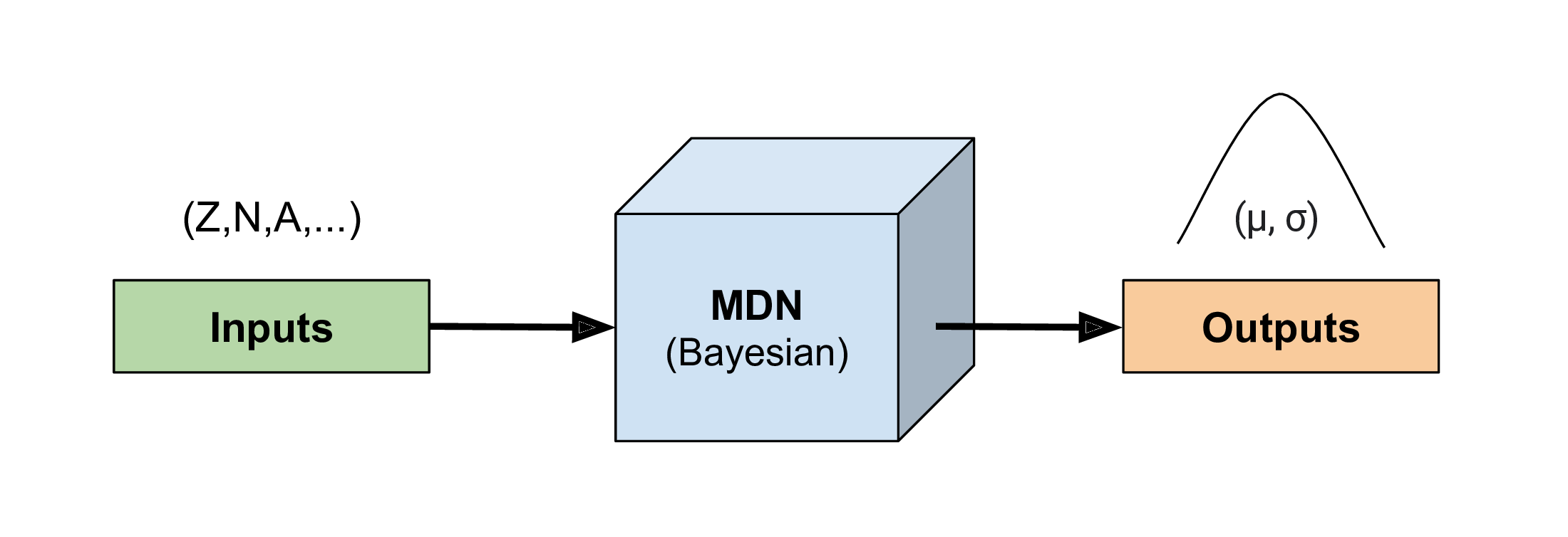We take a Bayesian approach: our network inputs are sampled based on nuclear data uncertainties

Our network outputs are therefore statistical

We can represent outputs by a collection of Gaussians (for masses we only need 1)

Our network therefore, also provides a quanitfied estimate of uncertainty (fully propagated through the model)

Lovell, Mohan, Sprouse & Mumpower submitted (2021)

## Results: predicting atomic masses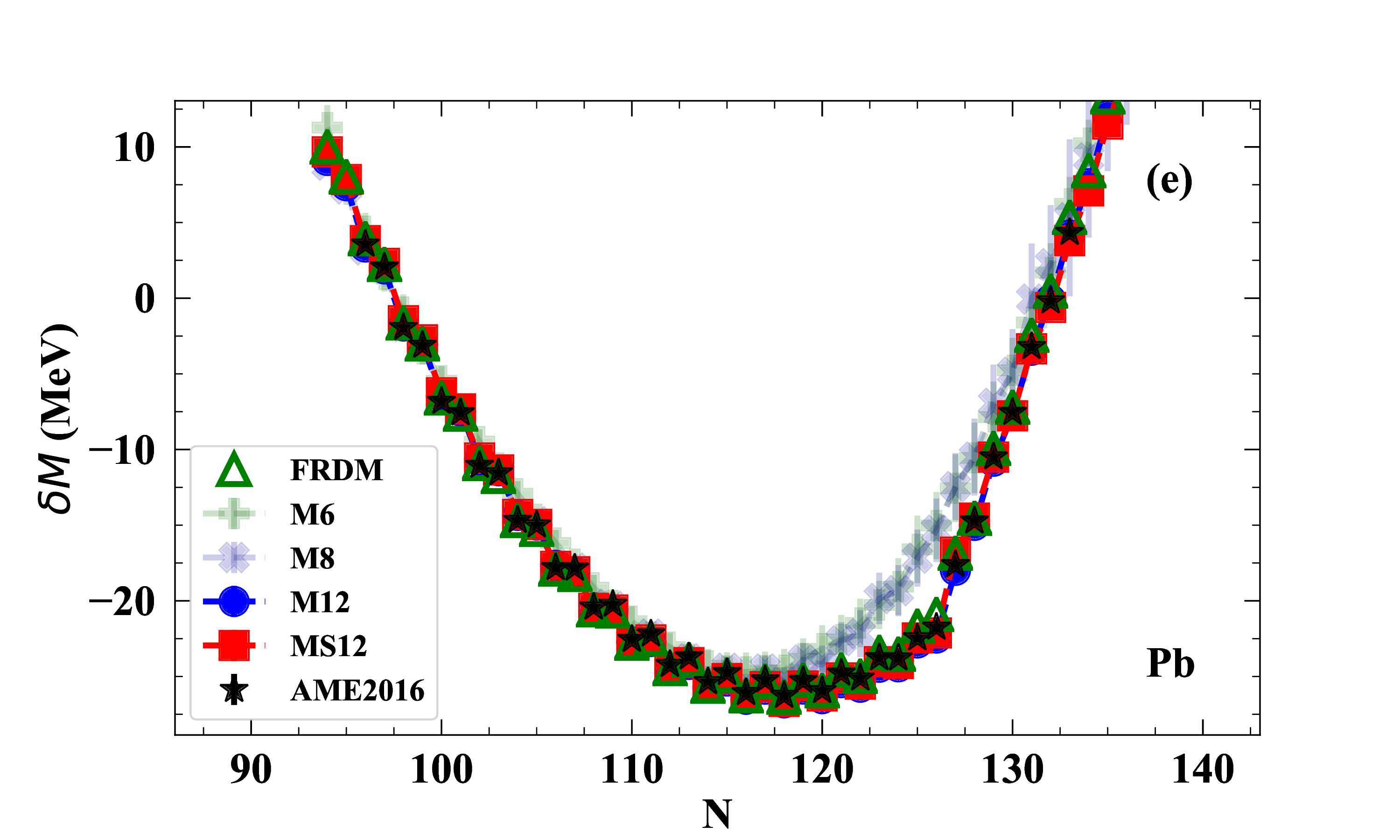Here we show results along the lead (Pb) [$Z=82$] isotopic chain

Data from the Atomic Mass Evaluation (AME2016) in black stars

Increasing sophistication of the model feature space indicated by M##

Lovell, Mohan, Sprouse & Mumpower submitted (2021)

## Results: predicting atomic masses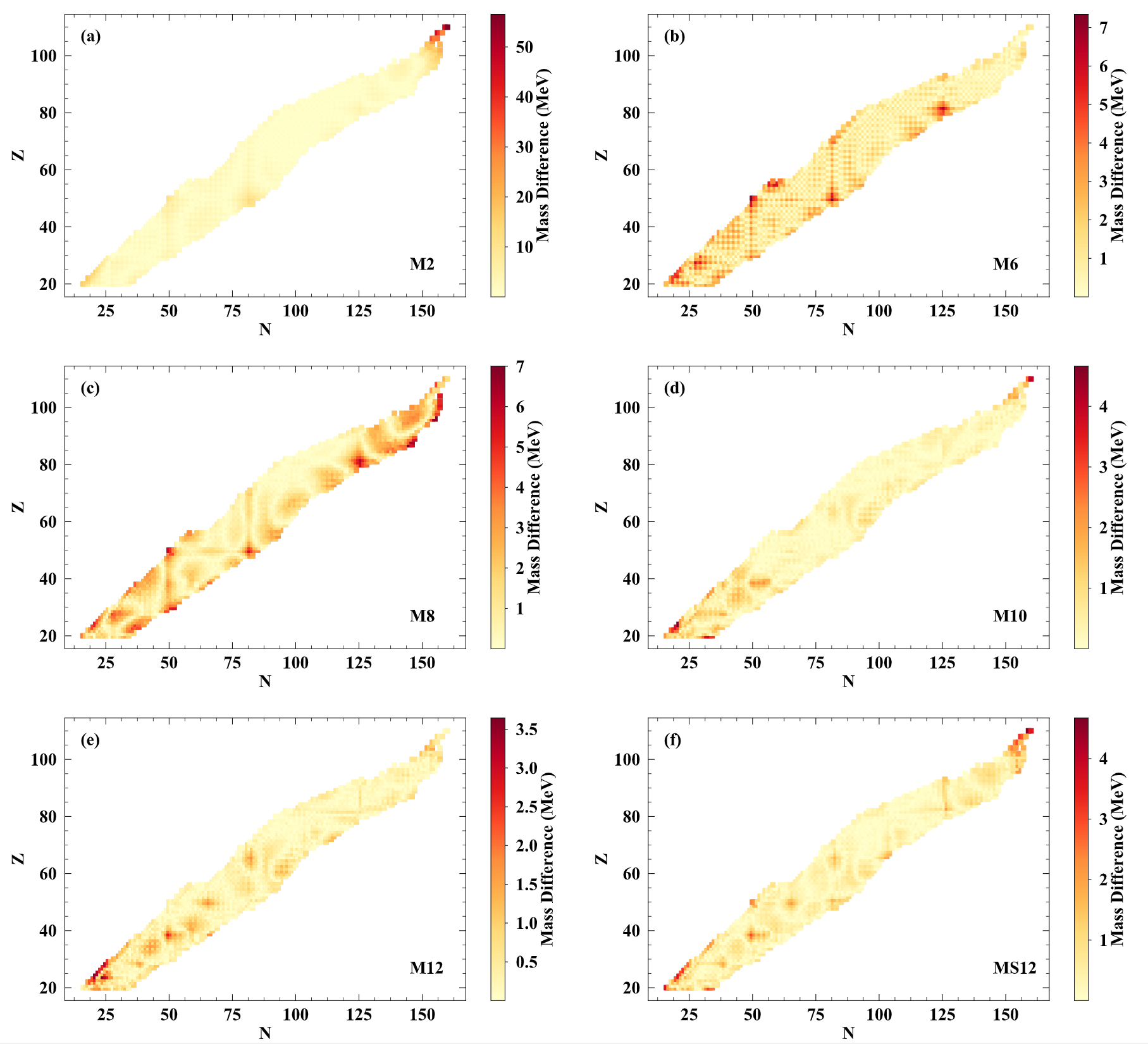Results across the chart of nuclides with increasing feature space complexity

Note the decrease in the maximum value of the scale

Lovell, Mohan, Sprouse & Mumpower submitted (2021)

## Results: predicting atomic masses

A summary of results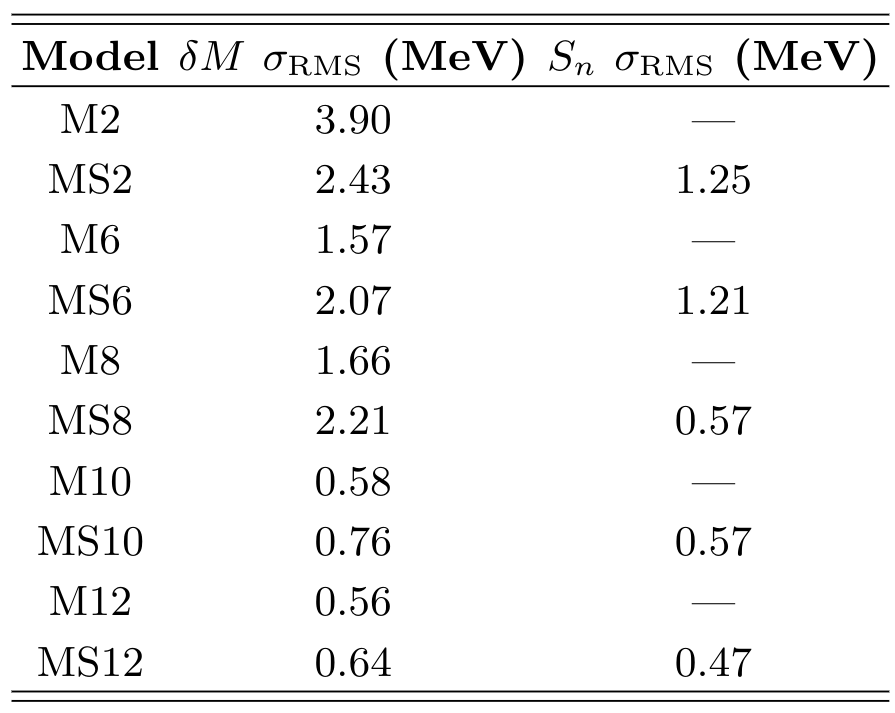Some lessons learned:

More physics added to the feature space → the better our description of atomic masses

Attempting to fit and predict other mass related quantities (e.g. separation energies) does impact our results (although marginal compared to choice of feature space)

We do not need deep neural networks to describe atomic masses; nor for extrapolations!

Lovell, Mohan, Sprouse & Mumpower submitted (2021)

## Special thanks to

My collaborators

A. Lovell, A. Mohan, & T. Sprouse

PostdocAnd to my son Zachary for the use of his drawings

## Summary

We are entering the era of computational nuclear data